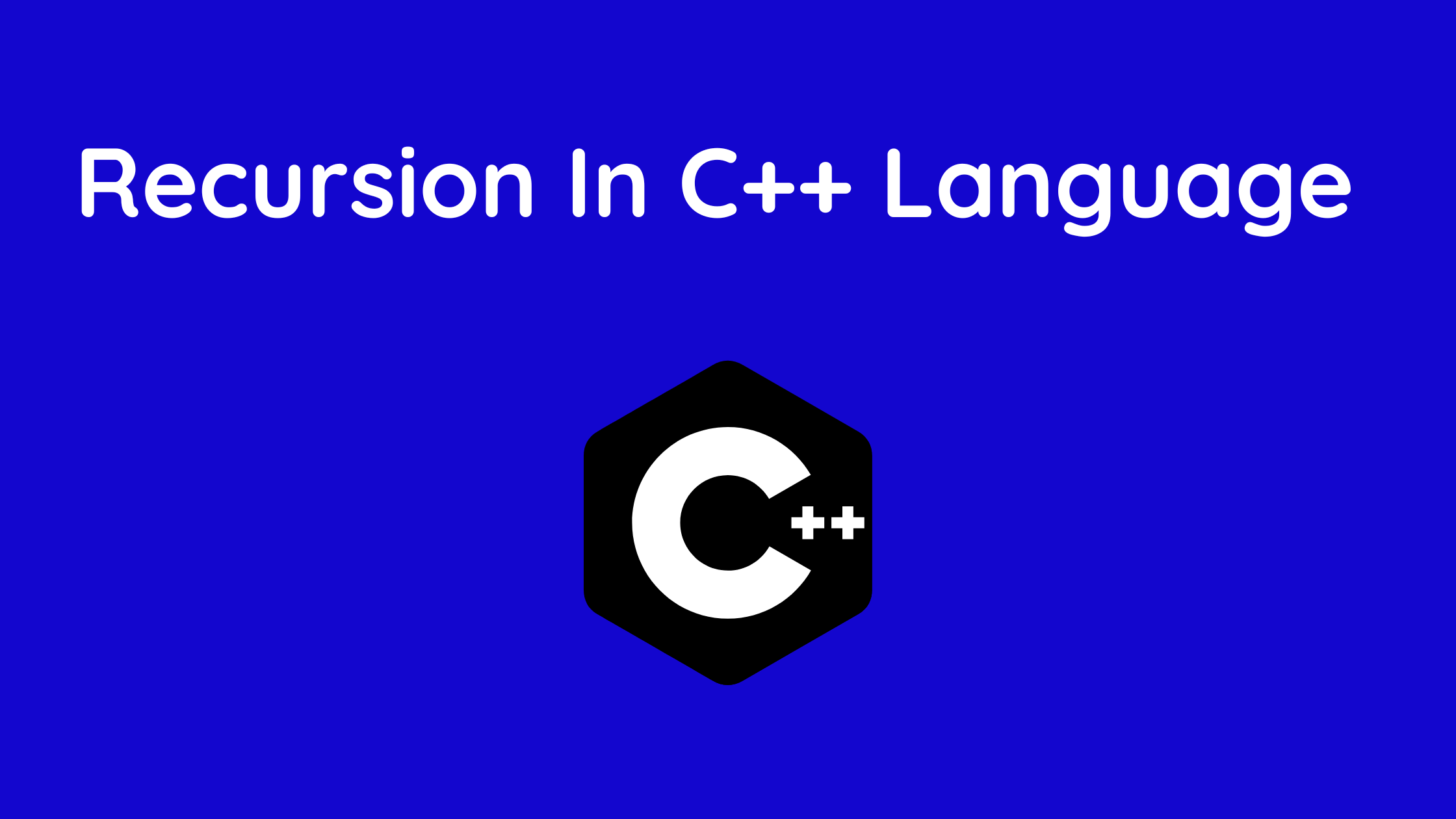## Recursion In C++ Language (Basic Recursion Example)

In this article, you will learn what is recursion with the help of an example. Recursion is the term used in C++ to describe calling a function from within another function. Recursive functions are those that repeatedly call the same function.

Recursion is the action of a function calling itself either directly or indirectly, and the associated function is known as a recursive function.

A recursive algorithm can be used to tackle some issues with relative ease.

Towers of Hanoi (TOH), inorder/preorder/postorder tree traversals, DFS of Graph, etc. are a few examples of these issues.

By calling a copy of itself and resolving the original problem’s smaller subproblems, a recursive function solves a specific problem. As and when necessary, many additional recursive calls can be produced.

It is crucial to understand that in order to stop this recursion process, we must present a specific situation.

Therefore, we can conclude that the function calls itself with a simplified version of the initial issue each time.

Tail recursion is the term for a function that just calls itself and doesn’t do anything else after the function call.

The same function is typically called with a return statement in tail recursion.

• With the aid of examples, we will learn about recursive functions in C++ and how they operate in this article.
• To begin with, we already understand the fundamental concept of C++ functions, which includes the ability to call other functions through function creation.
• Additionally, the recursive definition, a key idea in programming logic and mathematics, is covered in this article. One well-known illustration is the factorial of a number, the sum of ‘n’ natural numbers, etc.
• Recursive functions are defined as those that call themselves repeatedly. They are merely a function that is frequently called.
• Recursion provides a method for addressing difficulties that splits huge issues into manageable jobs that each work out individually to follow a separate sequence.
• Recursive functions are defined as those that call themselves repeatedly. And this method is called recursion.
• The recursive function is used as a solution for data structure ideas including searching, sorting, and tree traversal. This programming method simplifies the code.

Recursion differs from iteration in that it executes a specified section using the basic function itself, whereas iteration just repeats the code. We will go into detail about the significance of recursion and how it works with an example in this article.

Contents

## Recursion functionality in C++

```void recurse()
{
... .. ...
recurse();
... .. ...
}

int main()
{
... .. ...
recurse();
... .. ...
}```

The figure below demonstrates how recursion operates by repeatedly invoking itself.

Until a certain condition is met, the recursion continues.

When one branch performs the recursive call while the other does not, and if…else statement (or a similar approach) can be used to prevent infinite recursion.

Recursion repeats the function calls, and it comes to an end when the base case is realised. To prevent the stack overflow error message, the recursive function should declare a base case condition.

Infinite recursion results if no base case is specified. Each time a function is called, it pushes the resources reserved for subsequent calls into a stack. It provides the best tree traversal results.

Recursion comes in two flavours: direct recursion and indirect recursion.

## Example 1: Factorial of a Number Using Recursion

```// Factorial of n = 1*2*3*...*n

#include <iostream>
using namespace std;

int factorial(int);

int main() {
int n, result;

cout << "Enter a non-negative number: ";
cin >> n;

result = factorial(n);
cout << "Factorial of " << n << " = " << result;
return 0;
}

int factorial(int n) {
if (n > 1) {
return n * factorial(n - 1);
} else {
return 1;
}
}```

Output

Enter a non-negative number: 4
Factorial of 4 = 24

## Recursion’s benefits in c++

• It streamlines and shortens our code.
• In situations involving data structures and sophisticated algorithms, such as Graph and Tree Traversal, this is necessary.
• They simplified the more sophisticated, bulkier software to produce neat, compact code.
• fewer variables are used in the software code.
• Here, nested for loops and complex code are avoided.
• Backtracking is needed to solve a portion of the algorithm, which is done recursively.

## Recursion’s drawbacks in C++

Recursion In C++ Language, what is recursion, recursion in c, recursion in c++, Recursion In C++ Language, what is recursion, recursion in c, recursion in c++

• Compared to an iterative programme, it consumes a lot of stack space.
• It requires greater processing power.
• Compared to an analogous iterative programme, it may be more challenging to debug.
• due to the stack operation of all the function calls, requires greater memory allocation.
• It occasionally operates more slowly while carrying out the iteration process. As a result, efficiency is lower.
• Beginners may find it challenging to comprehend the work because the coding can occasionally be complex.
• if results in running out of space and eventually causes programme crashes.

https://theudaipurstore.com/maharana-pratap/